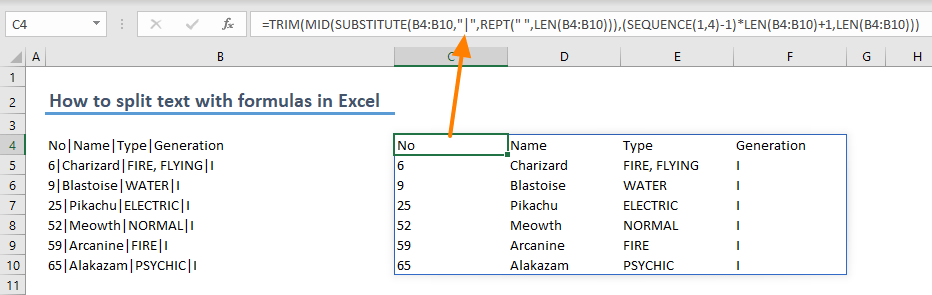Excel has more than one way for splitting text, such as Text to Columns feature. However, the feature requires a manual user intervention. You can make the process dynamic with help of functions. In this guide, we're going to show you how to split text with formulas in Excel.

Before start we should warn you that you should be a Microsoft 365 subscriber to use the following formula due to the SEQUENCE function and dynamic array feature.

## Data

We have rows of string containing data separated by “|” characters. Each part represents an individual column.## Syntax for splitting text with formulas

=TRIM(

MID(

SUBSTITUTE( text, separator, REPT( " ", LEN( text ))),

(SEQUENCE( 1, column count ) - 1 ) * LEN( text ) + 1,

LEN( text )))

## How it works

The formula relies on replacing separator character with a long enough space-character string which will act as cushion around the string. Let’s dive into details.

The SUBSTITUTE function replaces the separator with space character.

SUBSTITUTE(text, old_text, new_text, [instance_num])

Since any part of the whole string cannot include more characters than original string, the character count can be used to generate “long enough” padding string. This can be handled with the REPT function which simply repeats the given string a given number of times. The LEN function can provide the character count of the given text.

REPT(text, number_times)

LEN(text)

For example, “No|Name|Type|Generation” string becomes "No                       Name                       Type                       Generation" after transformation.

Once replacement takes place, each data can be separated into string groups thanks to the MID function. The MID function can parse a string from a text value based on a start point and character length.

MID(text, start_num, num_chars)

The trick behind calculating the starting point (start_num) and number of characters (num_chars) is using the length of full string. When the text with spaces is separated into chunks equal to original character length, each chunk will contain a data piece along with cushion spaces.

For example, "No                       Name                       Type                       Generation" string will be separated into

"No                     "

"  Name                 "

"      Type             "

"          Generation"

The calculation for calculating the star points includes the SEQUENCE function to generate numbers starting from 1 to maximum column number. The array returning from the SEQUENCE function causes the MID function return an array as well.

(SEQUENCE(1,column number)-1)*LEN(text)+1

If you want to calculate the column count dynamically, you can count the delimiter character in the rows and use that number in the formula: How to count a specific character in a string in Excel

Finally, the TRIM function removes the cushion space characters around the separated data.

TRIM(text)

## Example of splitting text with formulas

Thanks to the Excel’s dynamic array feature, you can handle multiple rows with a single data.

=TRIM(MID(SUBSTITUTE(B4:B10,"|",REPT(" ",LEN(B4:B10))),(SEQUENCE(1,4)-1)*LEN(B4:B10)+1,LEN(B4:B10)))### Bonus: LET Function version with dynamic columns

If you have the chance, check out the version with new LET function which reduces the references to deal with by defining in-formula named ranges. Although, the formula seems too complex at the first sight, it is just a version with name-reference pairs. If you want to use our splitting text with formulas approach on different places, this version will ease the process.

=LET(text,B14:B20,separator,"|",TRIM(MID(SUBSTITUTE(text,separator,REPT(" ",LEN(text))),(SEQUENCE(1,MAX(LEN(text)-LEN(SUBSTITUTE(text,separator,"")))+1)-1)*LEN(text)+1,LEN(text))))